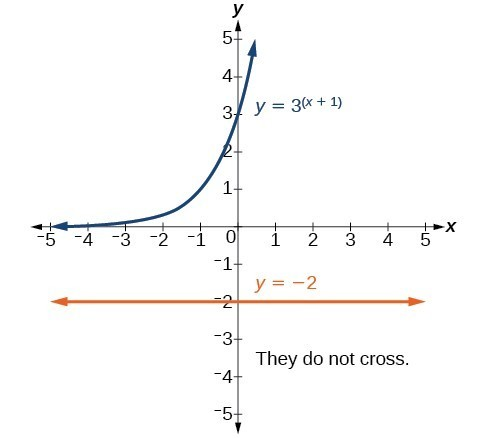## Exponential Equations with Like Bases

### Learning Outcomes

• Identify an exponential equation whose terms all have the same base
• Identify cases where equations can be rewritten so all terms have the same base
• Apply the one-to-one property of exponents to solve an exponential equation

When an exponential equation has the same base on each side, the exponents must be equal. This also applies when the exponents are algebraic expressions. Therefore, we can solve many exponential equations by using the rules of exponents to rewrite each side as a power with the same base. Then, we can set the exponents equal to one another and solve for the unknown.

For example, consider the equation ${3}^{4x - 7}=\frac{{3}^{2x}}{3}$. To solve for x, we use the division property of exponents to rewrite the right side so that both sides have the common base, $3$. Then we apply the one-to-one property of exponents by setting the exponents equal to one another and solving for x:

$\begin{array}{c}{3}^{4x - 7}\hfill & =\frac{{3}^{2x}}{3}\hfill & \hfill \\ {3}^{4x - 7}\hfill & =\frac{{3}^{2x}}{{3}^{1}}\hfill & {\text{Rewrite 3 as 3}}^{1}.\hfill \\ {3}^{4x - 7}\hfill & ={3}^{2x - 1}\hfill & \text{Use the division property of exponents}\text{.}\hfill \\ 4x - 7\hfill & =2x - 1\text{ }\hfill & \text{Apply the one-to-one property of exponents}\text{.}\hfill \\ 2x\hfill & =6\hfill & \text{Subtract 2}x\text{ and add 7 to both sides}\text{.}\hfill \\ x\hfill & =3\hfill & \text{Divide by 2}\text{.}\hfill \end{array}$

In our first example, we solve an exponential equation whose terms all have a common base.

### Example

Solve ${2}^{x - 1}={2}^{2x - 4}$.

In general, we can summarize solving exponential equations whose terms all have the same base in this way:
For any algebraic expressions S and T, and any positive real number $b\ne 1$

${b}^{S}={b}^{T}\text{ if and only if }S=T$
• Use the rules of exponents to simplify, if necessary, so that the resulting equation has the form ${b}^{S}={b}^{T}$.
• Use the one-to-one property to set the exponents equal to each other.
• Solve the resulting equation, = T, for the unknown.

## Rewriting Equations So All Powers Have the Same Base

Sometimes we can rewrite the terms in an equation as powers with a common base and solve using the one-to-one property. This takes a keen eye for recognizing common powers.  For example, you can rewrite 8 as $2^3$ or 36 as $6^2$ or $\frac{1}{4}$ as $\left(\frac{1}{2}\right)^{2}$

Consider the equation $256={4}^{x - 5}$. We can rewrite both sides of this equation as a power of $2$. Then we apply the rules of exponents, along with the one-to-one property, to solve for x:

$\begin{array}{c}256={4}^{x - 5}\hfill & \hfill \\ {2}^{8}={\left({2}^{2}\right)}^{x - 5}\hfill & \text{Rewrite each side as a power with base 2}.\hfill \\ {2}^{8}={2}^{2x - 10}\hfill & \text{Use the power to a power property of exponents}.\hfill \\ 8=2x - 10\hfill & \text{Apply the one-to-one property of exponents}.\hfill \\ 18=2x\hfill & \text{Add 10 to both sides}.\hfill \\ x=9\hfill & \text{Divide by 2}.\hfill \end{array}$
In the next example, we show how to find a common base for two expressions whose bases are $8$ and $16$. We can then solve the resulting equation using the one-to-one property of exponents.

### Example

Solve ${8}^{x+2}={16}^{x+1}$.

In our next example, we are given an exponential equation that contains a square root.  Remember that you can write roots as rational exponents, so you may be able to find like bases when it is not completely obvious at first.

### Example

Solve ${2}^{5x}=\sqrt{2}$.

By changing $\sqrt{2}$ to ${2}^{\frac{1}{2}}$, we were able to solve the equation in the previous example. In general, here are some steps to consider when you are solving exponential equations.  A good first step is always to determine whether you can rewrite the terms with a common base.

1. Rewrite each side of the equation as a power with a common base.
2. Use the rules of exponents to simplify, if necessary, so that the resulting equation has the form ${b}^{S}={b}^{T}$.
3. Use the one-to-one property to set the exponents equal to each other.
4. Solve the resulting equation, = T, for the unknown.

In the following video, we show more examples of how to solve exponential equations by finding a common base.

Do all exponential equations have a solution? If not, how can we tell if there is a solution during the problem-solving process? Write your thoughts in the textbox below before you check our proposed answer.

In the next example, we show you a case where there is no solution to an exponential equation. Remember how exponential functions are defined and ask yourself, “does this make sense”, before diving into solving exponential equations.

### Example

Solve ${3}^{x+1}=-2$.

### Analysis of the Solution

The figure below shows the graphs of the two separate expressions in the equation ${3}^{x+1}=-2$ as $y={3}^{x+1}$ and $y=-2$. The two graphs do not cross showing us that the left side is never equal to the right side. Thus the equation has no solution.## Summary

We can use the one-to-one property of exponents to solve exponential equations whose bases are the same.  The terms in some exponential equations can be rewritten with the same base, allowing us to use the same principle. There are exponential equations that do not have solutions because we define exponential functions as having a positive base. When restrictions are placed on the inputs of a function, it is natural that there will be restrictions on the output as well.